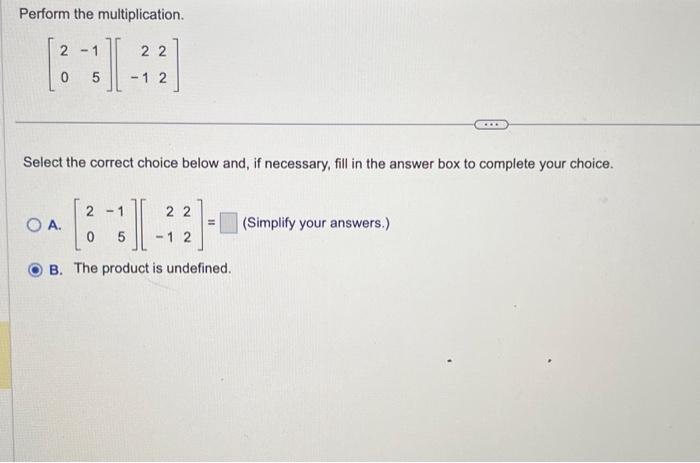Home / Expert Answers / Other Math / 12-perform-the-multiplication-left-begin-array-rr-2-1-0-5-end-array-right-left-b-pa727

# (Solved): 12 Perform the multiplication. $\left[\begin{array}{rr} 2 & -1 \\ 0 & 5 \end{array}\right]\left[\b ... 12Perform the multiplication. \[ \left[\begin{array}{rr} 2 & -1 \\ 0 & 5 \end{array}\right]\left[\begin{array}{rr} 2 & 2 \\ -1 & 2 \end{array}\right]$ Select the correct choice below and, if necessary, fill in the answer box to complete your choice. A. $$\left[\begin{array}{rr}2 & -1 \\ 0 & 5\end{array}\right]\left[\begin{array}{rr}2 & 2 \\ -1 & 2\end{array}\right]=$$ (Simplify your answers.) B. The product is undefined.

We have an Answer from Expert